The OpenFOAM Foundation
DiagTensor< Cmpt > Class Template Reference

Templated 3D DiagTensor derived from VectorSpace. More...

Inheritance diagram for DiagTensor< Cmpt >: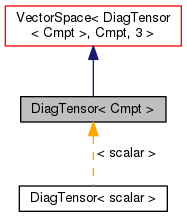[legend]
Collaboration diagram for DiagTensor< Cmpt >: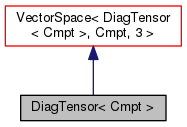[legend]

## Public Types

enum  components { XX, YY, ZZ }
Component labeling enumeration. More...

typedef DiagTensor< labellabelType
Equivalent type of labels used for valid component indexing. More...Public Types inherited from VectorSpace< DiagTensor< Cmpt >, Cmpt, 3 >
typedef VectorSpace< DiagTensor< Cmpt >, Cmpt, Ncmpts > vsType
VectorSpace type. More...

typedef Cmpt cmptType
Component type. More...

## Public Member Functions

DiagTensor ()
Construct null. More...

DiagTensor (const Foam::zero)
Construct initialized to zero. More...

template<class Cmpt2 >
DiagTensor (const VectorSpace< DiagTensor< Cmpt2 >, Cmpt2, 3 > &)
Construct given VectorSpace. More...

DiagTensor (const Cmpt &txx, const Cmpt &tyy, const Cmpt &tzz)
Construct given three components. More...

DiagTensor (Istream &)
Construct from Istream. More...

const Cmpt & xx () const

const Cmpt & yy () const

const Cmpt & zz () const

Cmpt & xx ()

Cmpt & yy ()

Cmpt & zz ()Public Member Functions inherited from VectorSpace< DiagTensor< Cmpt >, Cmpt, 3 >
VectorSpace ()
Construct null. More...

VectorSpace (const Foam::zero)
Construct initialized to zero. More...

VectorSpace (Istream &)
Construct from Istream. More...

VectorSpace (const VectorSpace< DiagTensor< Cmpt >, Cmpt, Ncmpts > &)
Construct as copy. More...

VectorSpace (const VectorSpace< Form2, Cmpt2, Ncmpts > &)
Construct as copy of a VectorSpace with the same size. More...

const Cmpt & component (const direction) const

Cmpt & component (const direction)

void component (Cmpt &, const direction) const

void replace (const direction, const Cmpt &)

const ConstBlock< SubVector, BStart > block () const

const Cmpt & operator[] (const direction) const

Cmpt & operator[] (const direction)

void operator= (const VectorSpace< DiagTensor< Cmpt >, Cmpt, Ncmpts > &)

void operator= (const Foam::zero)

void operator+= (const VectorSpace< DiagTensor< Cmpt >, Cmpt, Ncmpts > &)

void operator-= (const VectorSpace< DiagTensor< Cmpt >, Cmpt, Ncmpts > &)

void operator*= (const scalar)

void operator/= (const scalar)

## Static Public Attributes

static const direction rank = 2
Rank of DiagTensor is 2. More...Static Public Attributes inherited from VectorSpace< DiagTensor< Cmpt >, Cmpt, 3 >
static const direction dim
Dimensionality of space. More...

static const direction nComponents
Number of components in this vector space. More...

static const direction mRows

static const direction nCols

static const char *const typeName

static const char *const componentNames []

static const DiagTensor< Cmpt > zero

static const DiagTensor< Cmpt > one

static const DiagTensor< Cmpt > max

static const DiagTensor< Cmpt > min

static const DiagTensor< Cmpt > rootMax

static const DiagTensor< Cmpt > rootMinStatic Public Member Functions inherited from VectorSpace< DiagTensor< Cmpt >, Cmpt, 3 >
static direction size ()
Return the number of elements in the VectorSpace = Ncmpts. More...

static DiagTensor< Cmpt > uniform (const Cmpt &s)
Return a VectorSpace with all elements = s. More...Public Attributes inherited from VectorSpace< DiagTensor< Cmpt >, Cmpt, 3 >
Cmpt v_ [Ncmpts]
The components of this vector space. More...

## Detailed Description

### template<class Cmpt> class Foam::DiagTensor< Cmpt >

Templated 3D DiagTensor derived from VectorSpace.

Adding construction from 3 components, element access using xx(), yy() and zz() member functions and the inner-product (dot-product) and outer-product operators.

Source files

Definition at line 53 of file DiagTensor.H.

## Member Typedef Documentation

 typedef DiagTensor

Equivalent type of labels used for valid component indexing.

Definition at line 61 of file DiagTensor.H.

## Member Enumeration Documentation

 enum components

Component labeling enumeration.

Enumerator
XX
YY
ZZ

Definition at line 71 of file DiagTensor.H.

## Constructor & Destructor Documentation

 DiagTensor ( )
inline

Construct null.

Definition at line 32 of file DiagTensorI.H.

Referenced by DiagTensor< Cmpt >::DiagTensor().

Here is the caller graph for this function: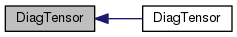DiagTensor ( const Foam::zero )
inline

Construct initialized to zero.

Definition at line 37 of file DiagTensorI.H.

References DiagTensor< Cmpt >::DiagTensor().

Here is the call graph for this function: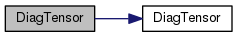DiagTensor ( const VectorSpace< DiagTensor< Cmpt2 >, Cmpt2, 3 > & vs )
inline

Construct given VectorSpace.

Definition at line 46 of file DiagTensorI.H.

References DiagTensor< Cmpt >::DiagTensor().

Here is the call graph for this function:DiagTensor ( const Cmpt & txx, const Cmpt & tyy, const Cmpt & tzz )
inline

Construct given three components.

Definition at line 56 of file DiagTensorI.H.

 DiagTensor ( Istream & is )
inline

Construct from Istream.

Definition at line 69 of file DiagTensorI.H.

## Member Function Documentation

 const Cmpt & xx ( ) const
inline

Definition at line 78 of file DiagTensorI.H.

Here is the caller graph for this function: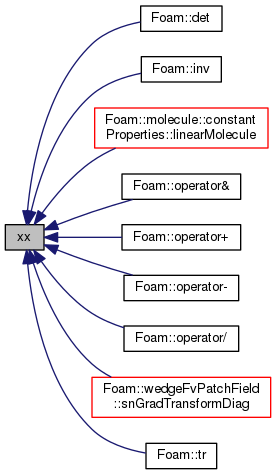const Cmpt & yy ( ) const
inline

Definition at line 84 of file DiagTensorI.H.

Here is the caller graph for this function: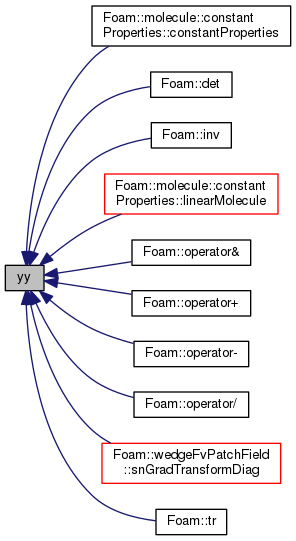const Cmpt & zz ( ) const
inline

Definition at line 90 of file DiagTensorI.H.

Here is the caller graph for this function: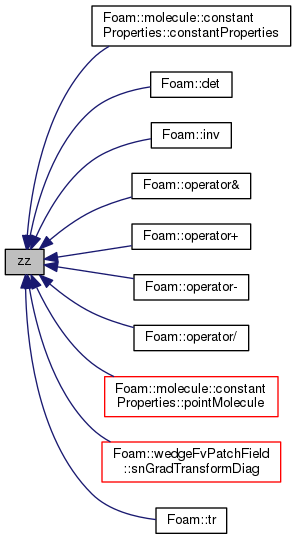Cmpt & xx ( )
inline

Definition at line 97 of file DiagTensorI.H.

 Cmpt & yy ( )
inline

Definition at line 103 of file DiagTensorI.H.

 Cmpt & zz ( )
inline

Definition at line 109 of file DiagTensorI.H.

## Member Data Documentation

 const direction rank = 2
static

Rank of DiagTensor is 2.

Definition at line 67 of file DiagTensor.H.

The documentation for this class was generated from the following files: